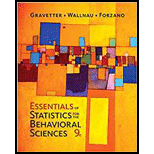Chapter 5, Problem 7P### Essentials of Statistics for The B...

9th Edition
Frederick J Gravetter + 2 others
ISBN: 9781337098120

#### Solutions

Chapter
Section### Essentials of Statistics for The B...

9th Edition
Frederick J Gravetter + 2 others
ISBN: 9781337098120
Textbook Problem

# A sample has a mean of M =60 and a standard devia­tion of s = 7. For this sample, find the z-score for each of the following X values.X = 69        X = 72        X = 63X = 54        X = 49        X = 52

To determine

To Find: The z score corresponding to X.

Explanation

Given Info:

The sample has mean M=60 and standard deviation s=7.

Calculation:

The formula for z score is,

z=XMs

The z score corresponding to X=69 is,

z=XMs=69607=1.29

The z score corresponding to X=72 is,

z=XMs=72607=1.71

The z score corresponding to X=63 is,

z=XMs=63607=0

### Still sussing out bartleby?

Check out a sample textbook solution.

See a sample solution

#### The Solution to Your Study Problems

Bartleby provides explanations to thousands of textbook problems written by our experts, many with advanced degrees!

Get Started

## Additional Math Solutions

#### Evaluate the expression sin Exercises 116. 22212424

Finite Mathematics and Applied Calculus (MindTap Course List)

#### let f(x) = x3 + 5, g(x) = x2 2, and h(x)= 2x + 4. Find the rule for each function. 2. f g

Applied Calculus for the Managerial, Life, and Social Sciences: A Brief Approach

#### True or False: converges.

Study Guide for Stewart's Multivariable Calculus, 8th